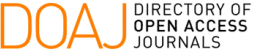### An Efficient Quantum Algorithm for the Hidden Subgroup Problem over some Non-Abelian Groups

Demerson Nunes Gonçalves, Tharso D Fernandes, C M M Cosme

#### Abstract

The hidden subgroup problem (HSP) plays an important role in quantum computation, because many quantum algorithms that are exponentially faster than classical algorithms are special cases of the HSP. In this paper we show that there exist a new efficient quantum algorithm for the HSP on groups \$\Z_{N}\rtimes\Z_{q^s}\$ where \$N\$ is an integer with a special prime factorization, \$q\$ prime number and \$s\$ any positive integer.

#### Keywords

Quantum Algorithms, Hidden Subgroup Problem, Quantum Computational Group Theory

PDF

#### References

R. Beals. “Quantum computation of Fourier transforms over symmetric groups”. Proc. 29th ACM Symp. on Theory of Computing, pages 48–53, New York, (1997).

D. Boneh & R.J. Lipton. “Quantum cryptanalysis of hidden linear functions”, In Lecture Notes in Computer Science, volume 963, pages 424–437, Berlin, (1995).

W. Burniside. Theory of Groups of Finite order, Dover Publication, Inc. – New York, (1955).

K.K.H. Cheung & M. Mosca. Decomposing Finite Abelian Groups. Quantum Information and Computation, 1(3) (2001), 23–32.

D.P. Chi, J.S. Kim & S. Lee. Quantum algorithms for the hidden subgroup problem on some semi-direct product groups by reduction to Abelian cases. Physics Letters A, pages 114–116, (2006).

C.M.M. Cosme. “Algoritmos Quânticos para o Problema do Subgrupo Oculto não Abeliano”. Tese de Doutorado, LNCC, Petrópolis, RJ, (2008).

W. van Dam & S. Dey. “Hidden Subgroup Quantum Algorithms for a Class of Semi-Direct Product Groups”. Proc. of 9th Conference on the Theory of Quantum Computation, Communication and Cryptography, TQC’14, pages 110–117, (2014).

M. Ettinger & P. Høyer. “A quantum observable for the graph isomorphism problem”, arXiv:quant-ph/9901029, (1999).

A. Garcia & Y. Lequain. Elementos de A ́ lgebra, 3.ed. Rio de Janeiro: IMPA, 325 p. (Projeto Euclides), (2005).

D.N. Gonc ̧alves, R. Portugal & C.M.M. Cosme. “Solutions to the hidden subgroup problem on some metacylic groups”. Proc. 4th Worshop on Theory of Quantum Computation, Communication and Cryptography, LNCS, Springer-Verlag, (2009).

D.N. Gonc ̧alves & R. Portugal. “Solution to the Hidden Subgroup Problem for a Class of Noncom- mutative Groups”. Quantum Physics, Abstract quant-ph/1104.1361, (2011).

P. Hoyer. “Efficient quantum transforms”. arXiv:quant-ph/9702028, (1997).

Y. Inui & F. Le Gall. An efficient quantum algorithm for the hidden subgroup problem over a class of semi-direct product groups. Quantum Information and Computation, (2005).

C. Lomont. “The Hidden Subgroup Problem – Review and Open Problems”. Quantum Physics, Abstract quant-ph/0411037, (2004).

M. Mosca. “Quantum algorithms”. Encyclopedia of Complexity and Systems Science, pages 7088– 7118, (2009).

O. Regev. Quantum Computation and Lattice Problems. SIAM Journal on Computing, 33(3) (2004), 738–760.

D.R. Simon. “On the Power of Quantum Computation”. Proceedings of the 35th Annual Symposium on Foundations of Computer Science, pages 116–123, (1994).

P.W. Shor. “Algorithms for quantum computation: discrete logs and factoring”. Proc. of the 35th Ann. IEEE Symp. on the Foundation of Computer Science, pages 124–134, (1994).

DOI: https://doi.org/10.5540/tema.2017.018.02.0215

### Refbacks

• There are currently no refbacks.

Trends in Computational and Applied Mathematics

A publication of the Brazilian Society of Applied and Computational Mathematics (SBMAC)

Indexed in: